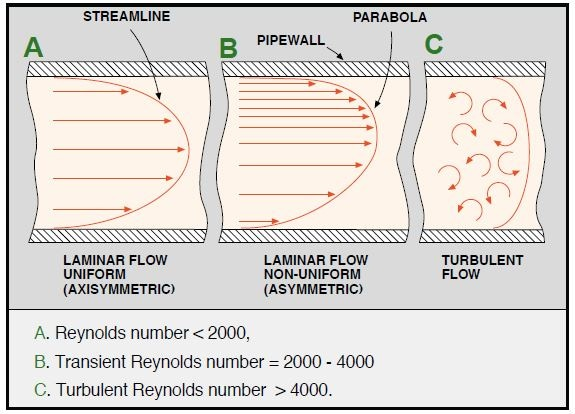# An Introduction to Flow Measurement & Fluid Properties

The many applications of flow measurement include uses in water supply, medical research, oil exploration, food processing and distribution of gas. The uses of this technology are very diverse, with each different situation bringing unique challenges and requirements.

OMEGA provides current and developing flow technologies which drive both industry and industrial processes. They help to improve these processes, working to make them cost-effective and efficient, while considering the time constraints of their customers.

## What Is a Flowmeter?

From within a pipe, both the rate of flow and the abundance of a gas or liquid can be measured with a device called a flow meter.

## How Can You Quantify Flow Rate?

The majority of flow measurement devices use velocity of the substance to determine the flow rate. This velocity is determined by the pressure differential which is pushing the liquid or gas through the pipeline. Since the circumference of the pipe can be measured and remains the same, the average velocity can be used to indicate the flow rate.

The equation used to calculate flow rate is: Q = V x A.

Q = flow rate (SI Unit m3/s)

V = average velocity (SI Unit m/s)

A = circumference of the pipe (SI Unit m2)

The density and friction of the fluid in contact with the pipe are the additional factors which may affect rate of flow.

## How to Predict Fluid Behavior

A vital number used in the prediction of flow patterns within differing situations is the Reynold number (Re). Re determines if the flow of either a liquid or gas is turbulent, laminar or transient. It is defined as a ratio between the liquid’s inertial force vs. its drag force.

Low Reynolds numbers indicate a laminar flow whereas high Reynolds numbers indicate a turbulent flow, due to contrasting speeds and directions. This turbulent flow can interest and alter the course of the flow.

The equation for the Reynolds numbers is: Re = (ρ x v x d)/µ

Re =Reynolds Number

ρ = fluid density (kg/m3)

v = mean flow velocity (m/s)

d = diameter of the pipe (m)

µ = viscosity of fluid (Pa.s)

The two main types of flow observed in these measurements, as represented in the diagram below, are laminar and turbulent flow. The large majority is turbulent flow, with Re values of approximately 4000. However, the flow in more viscous liquids is more frequently laminar flow, with Re values less than 2000.Increased flow turbulence and advanced flow profile development are two factors which improve the accuracy of measurements. The flow profile can be improved by reviewing installation requirements, particularly regarding the number of vertical pipes required after disruption, usually caused by either a bend or a valve placed before the flowmeter.

Gases tend to need more vertical pipelines to establish an accurate measurement in comparison to liquids. Therefore consideration of the installation requirements, the measurement objectives and the requirements will ensure a successful measurement.This information has been sourced, reviewed and adapted from materials provided by OMEGA Engineering Ltd.

## Citations

• APA

OMEGA Engineering Ltd. (2019, July 24). An Introduction to Flow Measurement & Fluid Properties. AZoM. Retrieved on October 28, 2020 from https://www.azom.com/article.aspx?ArticleID=15679.

• MLA

OMEGA Engineering Ltd. "An Introduction to Flow Measurement & Fluid Properties". AZoM. 28 October 2020. <https://www.azom.com/article.aspx?ArticleID=15679>.

• Chicago

OMEGA Engineering Ltd. "An Introduction to Flow Measurement & Fluid Properties". AZoM. https://www.azom.com/article.aspx?ArticleID=15679. (accessed October 28, 2020).

• Harvard

OMEGA Engineering Ltd. 2019. An Introduction to Flow Measurement & Fluid Properties. AZoM, viewed 28 October 2020, https://www.azom.com/article.aspx?ArticleID=15679.

## Tell Us What You Think